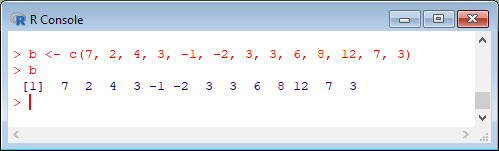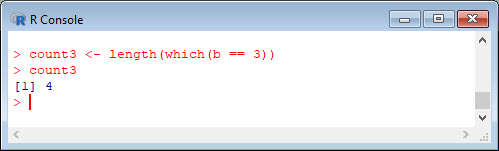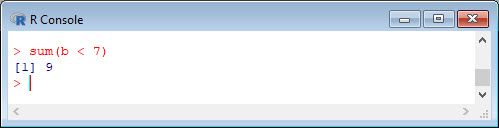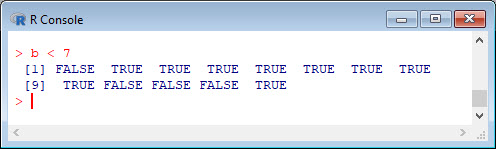##### Counting elements in a dataset

Combining the `length()` and `which()` commands gives a handy method of counting elements that meet particular criteria.
`b <- c(7, 2, 4, 3, -1, -2, 3, 3, 6, 8, 12, 7, 3) `
`b`Let’s count the 3s in the vector `b`.
`count3 <- length(which(b == 3)) `
`count3`In fact, you can count the number of elements that satisfy almost any given condition.
`length(which(b < 7))`Here is an alternative approach, also using the `length()` command, but also using square brackets for sub-setting:
`length(b[ b < 7 ])`##### R provides another alternative that not everyone knows about

`sum(b < 7)`This syntax gives a count rather than a sum. Be aware of the meaning of syntax like `sum(b < 7)`. Both work on logical vectors whose elements are either `TRUE` or `FALSE`. Try entering `b < 7` at the keyboard.
`b < 7`We see that `sum(b < 7)` counts the number of elements that are `TRUE`. There are nine such elements.
Now try:
`mean(b < 7)`That syntax found the proportion of elements meeting the criterion rather than the mean. Again, if you use the `sum()` and `mean()` function you must be very careful to ensure that your output is what you intended. Note that `sum()`, `length()` and `length(which())` all provide mechanisms for counting elements.
Now find the percentage of 7s in `b`.
`P7 <- 100 * length(which(b == 7)) / length(b) `
`P7`##### Extension example

You can find counts and percentages using functions that involve `length(which())`. Here we create two functions; one for finding counts, and the other for calculating percentages.
`count <- function(x, n){ length((which(x == n))) } `
`perc <- function(x, n){ 100*length((which(x == n))) / length(x) }`
Note the syntax involved in setting up a function in R. Now let’s use the `count()` function to count the threes in the vector `b` and the `perc()` function to calculate percentage of 4s in `b`.
```count(b, 3) perc(b, 4)```That wasn’t so hard! In Blog 18 I will present another tip for data analysis in R.
See you later!
David

#### Annex: R codes used

```# Create a vector b.
b <- c(7, 2, 4, 3, -1, -2, 3, 3, 6, 8, 12, 7, 3)
b

# Count the 3s in the vector b.
count3 <- length(which(b == 3))
count3

# Another example of counting.
length(which(b < 7))

# An alternative approach to counting.
length(b[ b < 7 ])

# Another alternative approach to counting
sum(b < 7)
b < 7

# Proportion of elements in b less than 7
mean(b < 7)

# Percentage of 7s in b.
P7 <- 100 * length(which(b == 7)) / length(b)
P7

# Create two functions count and percentage.
count <- function(x, n){ length((which(x == n))) }
perc <- function(x, n){ 100*length((which(x == n))) / length(x) }
count(b, 3)
perc(b, 4)
```